NEET  >  Test: Optical Isomerism

# Test: Optical Isomerism

Test Description

## 20 Questions MCQ Test Chemistry Class 11 | Test: Optical Isomerism

Test: Optical Isomerism for NEET 2023 is part of Chemistry Class 11 preparation. The Test: Optical Isomerism questions and answers have been prepared according to the NEET exam syllabus.The Test: Optical Isomerism MCQs are made for NEET 2023 Exam. Find important definitions, questions, notes, meanings, examples, exercises, MCQs and online tests for Test: Optical Isomerism below.
Solutions of Test: Optical Isomerism questions in English are available as part of our Chemistry Class 11 for NEET & Test: Optical Isomerism solutions in Hindi for Chemistry Class 11 course. Download more important topics, notes, lectures and mock test series for NEET Exam by signing up for free. Attempt Test: Optical Isomerism | 20 questions in 30 minutes | Mock test for NEET preparation | Free important questions MCQ to study Chemistry Class 11 for NEET Exam | Download free PDF with solutions
 1 Crore+ students have signed up on EduRev. Have you?
Test: Optical Isomerism - Question 1

### The number of optically active optical isomers of the compound is: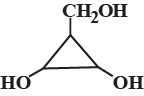Detailed Solution for Test: Optical Isomerism - Question 1

The number of optically active isomers = 2n−1−2n−1/2 ​=23−1−23−1/2 ​=2.

Test: Optical Isomerism - Question 2

### Find the number of stereoisomers for CH3 – CHOH – CH = CH – CH3.

Detailed Solution for Test: Optical Isomerism - Question 2

The number of stereoisomers for CH3 – CHOH – CH = CH – CH3 is four. This is calculated by the formula 2n+1. where n is number of chiral centres, which is 1 in this case. So, 21+1 = 22= 4

Test: Optical Isomerism - Question 3

### A hydrocarbon X is optically. X upon hydrogenation gives an optically inactive alkane Y. Which of the following pair of compounds can be X and Y respectively?

Detailed Solution for Test: Optical Isomerism - Question 3

The correct answer is Option B.
The optically active hydrocarbon X is 3-methyl-1-pentene CH2=CH−CH(CH3)CH2CH3​. On catalytic hydrogenation, it forms 3-methyl pentane CH3CH2CH(CH3)CH2CH3, which is optically inactive.

Test: Optical Isomerism - Question 4

What is wrong about enantiomers of 2-chloropropanoic acid?

Detailed Solution for Test: Optical Isomerism - Question 4

The correct answer is option D.
Pair of enantiomers react differently with pure enantiomers of other compounds.

Test: Optical Isomerism - Question 5

How many different stereoisomers exist for the compound below ?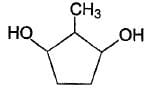Detailed Solution for Test: Optical Isomerism - Question 5

If you name the C attached to Me group as C1 and naming the rest clockwise, you observe that at C1 and C2 there are chiral centers and C5 and C2 are symmetrical. So the two chiral centers produce 2×2=4 optically active(and distinguishable) compounds. Hence, B is the answer.

Test: Optical Isomerism - Question 6

How many total cyclic isomers are possible for C5H10 ?

Detailed Solution for Test: Optical Isomerism - Question 6

The correct answer is Option B.

5 isomers are possible. C5H10(CnH2n). Molecules having the CnH2n formulas are most likely to be cyclic alkanes. The five isomers are: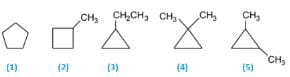(1)   Cyclopentane
(2) 1-Methylcyclobutane
(3) 1-Ethylcyclopropane
(4) 1,1-Dimethylcyclopropane
(5) 1,2-Dimethylcyclopropane

Test: Optical Isomerism - Question 7

How the following pair of isomers cannot be distinguished ?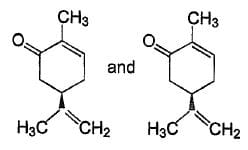Test: Optical Isomerism - Question 8

Which compound below exhibit stereolsomerism?

Detailed Solution for Test: Optical Isomerism - Question 8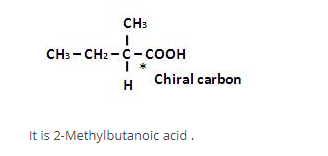Test: Optical Isomerism - Question 9

Consider all possible isomeric ketones, including stereoisomers of Molecular weight 100. All these isomers are independently reacted with NaBH4
(Note stereoisomers are also reacted separately). The total number of ketones that give a racemic product(s) is/are

Detailed Solution for Test: Optical Isomerism - Question 9

The general formula of isomeric ketone having molecular mass 100 is C6​H12​O [6×12+12×1+16].

The possible structure will be :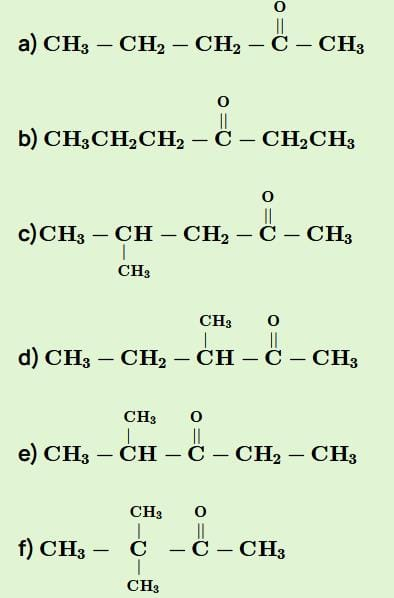The number of ketones that gives racemic mixture with NaBH4​ is 5 as the ketone with chiral center will give diastereoisomer with NaBH4​

*Multiple options can be correct
Test: Optical Isomerism - Question 10

Direction (Q. Nos. 10-14) This section contains 5 multiple choice questions. Each question has four
choices (a), (b), (c), and (d), out of which ONE or  MORE THAN ONE  is correct.

Q.Consider the following molecule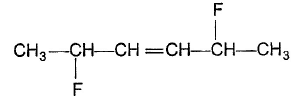The correct statement concerning the above molecule is/are

Detailed Solution for Test: Optical Isomerism - Question 10
•  The given compound has a total of six stereoisomers
• It's meso form upon ozonolysis followed by Zn-hydrolysis gives racemic mixture

• It's optically active isomers, each upon ozonolysis followed by Zn-hydrolysis gives a single enantiomer.

*Multiple options can be correct
Test: Optical Isomerism - Question 11

Consider the following free radical chlorination reaction.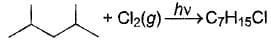The correct statement about product(s) is/are

Detailed Solution for Test: Optical Isomerism - Question 11

There are 4 final products, they are as follow:-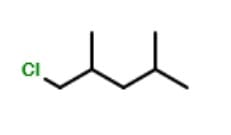2 products of the structure above due to presence of a chiral carbon.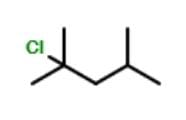1 product of structure above due to absence of Chiral Carbon.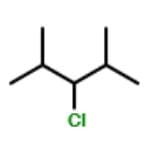1 product of structure above due to absence of Chiral Carbon.

Hence, Options A, B are correct.

*Multiple options can be correct
Test: Optical Isomerism - Question 12

The correct statement(s) regarding Isomers of a compound with general name trichlorocyclobutane is/are

Detailed Solution for Test: Optical Isomerism - Question 12
• Trichlorocyclobutane has total seven isomers
• It has two meso isomers
• One isomer is devoid of any chiral carbon
*Multiple options can be correct
Test: Optical Isomerism - Question 13

The correct statement (s) about the compound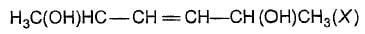is/are

[IIT JEE 2009]

Detailed Solution for Test: Optical Isomerism - Question 13

The correct options are A & D
Since a carbon-carbon double bond is present, the molecule can have either cis isomer or trans isomer.
In cis isomer (which is symmetrical), the number of enantiomers is
2(n−1)=2(2−1)=2
The number of meso compounds possible is 2(n/2)−1=2(2/2)−1=1
Hence, the total number of optical isomers possible is 2+1=3.
Trans isomer is also symmetrical.
Hence, the total number of optical isomers possible for trans isomers is also 3.
Hence, the total number of stereoisomers possible for X is 3+3=6.
Hence, option A is correct and option B is incorrect.
If the stereochemistry about the double bond in X is trans, the number of enantiomers possible for X is 4.
Thus, the option C is incorrect.
If the stereochemistry about the double bond in X is cis, the number of enantiomers possible for X is 2.
Thus the option D is correct.

*Multiple options can be correct
Test: Optical Isomerism - Question 14

The correct statement(s) about the compound given below is/are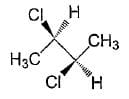Detailed Solution for Test: Optical Isomerism - Question 14

The correct answer is option A & D
The given image is Self-Explanatory.
We know that following are the cases when a compound is Optically Inactive:
1. When it does not have a chiral Carbon Atom eg.: Methyl bromide
2. When it has a Plane or Axis of Symmetry eg.: Tartaric Acid (these compounds are called as Meso Compounds)
3. When it forms a Racemic Mixture eg.: Equimolar mixture of d-Lactic Acid and l-Lactic Acid.
The given compound in the question possesses an Axis of Symmetry (shown in the attached image). Hence, it is Optically Inactive.

*Multiple options can be correct
Test: Optical Isomerism - Question 15

Which of the given statement (s) about N, O, P, and Q with respect to M is (are) correct?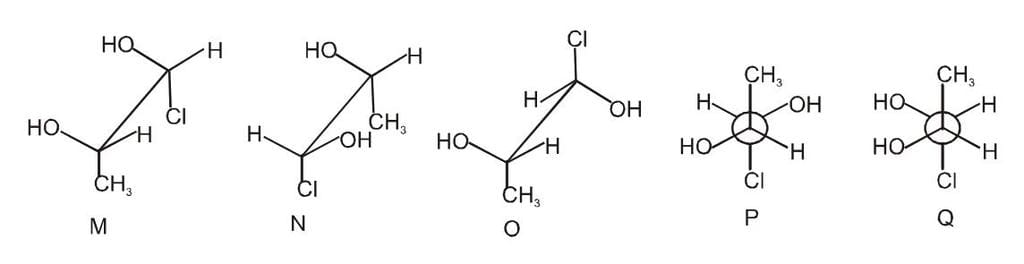Detailed Solution for Test: Optical Isomerism - Question 15

The Fischer Projections of the given compounds are below.

• M and N are non-mirror image stereoisomers
• M and O are identical
• M and P are enantiomers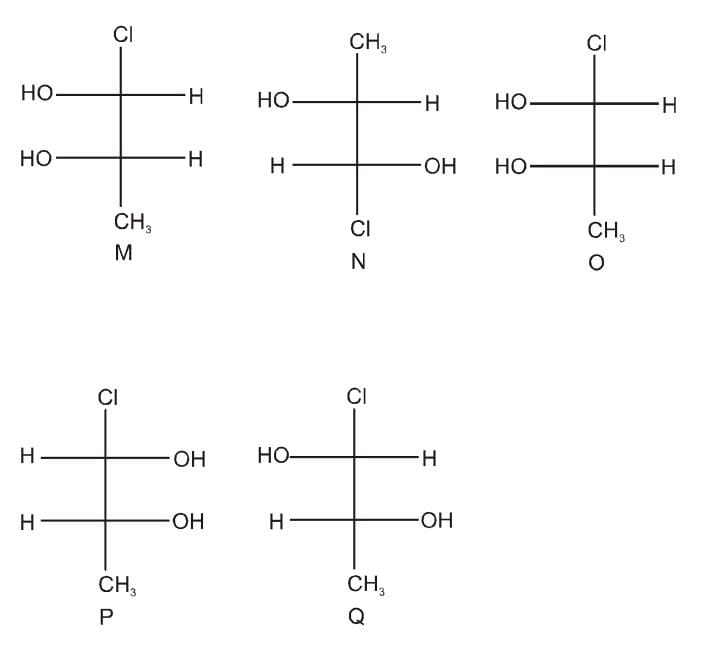Test: Optical Isomerism - Question 16

The number of optical isomers possible for 2, 3-pentanediol is:

Detailed Solution for Test: Optical Isomerism - Question 16

2 optical centers.
Total optically active isomers =22
=4

Test: Optical Isomerism - Question 17

The minimum number of C atoms required for a hydrocarbon to exhibit optical isomerism:

Detailed Solution for Test: Optical Isomerism - Question 17

The minimum number of C atoms required for a hydrocarbon to exhibit optical isomerism = 7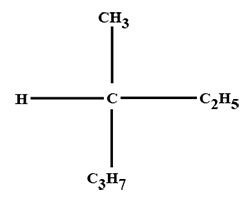*Multiple options can be correct
Test: Optical Isomerism - Question 18

Which of the following statements is/are correct regarding the below compound?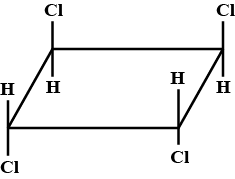Detailed Solution for Test: Optical Isomerism - Question 18

Correct option is
A:
It is optically inactive due to the plane of symmetry &

B: It is optically inactive due to the center of symmetry

The presence of any kind of symmetry makes the compound optically inactive.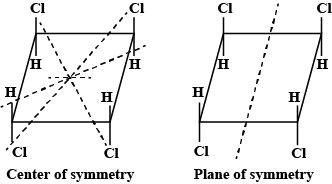Test: Optical Isomerism - Question 19

Which of the following are not functional isomers of each other?

Detailed Solution for Test: Optical Isomerism - Question 19

Functional isomers are structural isomers that have the same molecular formula (that is, the same number of atoms of the same elements), but the atoms are connected in different ways so that the groupings are dissimilar or different functional groups.

10 amine to different 10 amines are not functional isomers.

Hence, option ′d′ is the answer.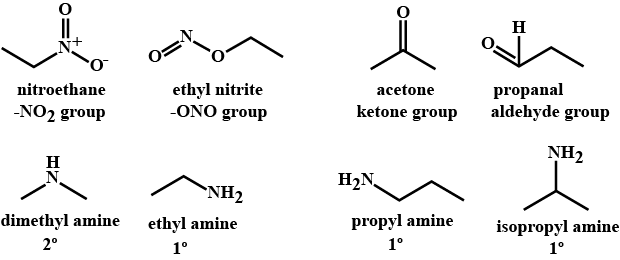Test: Optical Isomerism - Question 20

A pair of enantiomers is possible for _______ isomer of 2,2-dibromobicyclo [2.2.1] heptane.

Detailed Solution for Test: Optical Isomerism - Question 20

Only one pair of enantiomers is possible for cis-2,2-dibromobicyclo [2.2.1] heptane. The trans arrangement of one carbon bridge is structurally impossible. Such a molecule would have too much strain.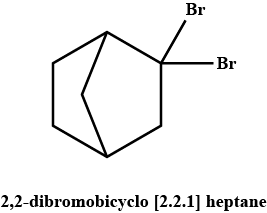## Chemistry Class 11

204 videos|331 docs|229 tests
 Use Code STAYHOME200 and get INR 200 additional OFF Use Coupon Code
Information about Test: Optical Isomerism Page
In this test you can find the Exam questions for Test: Optical Isomerism solved & explained in the simplest way possible. Besides giving Questions and answers for Test: Optical Isomerism, EduRev gives you an ample number of Online tests for practice

## Chemistry Class 11

204 videos|331 docs|229 tests

### How to Prepare for NEET

Read our guide to prepare for NEET which is created by Toppers & the best Teachers